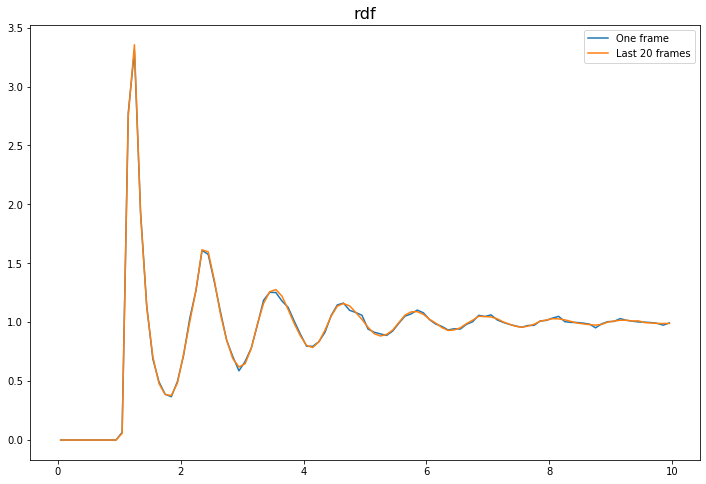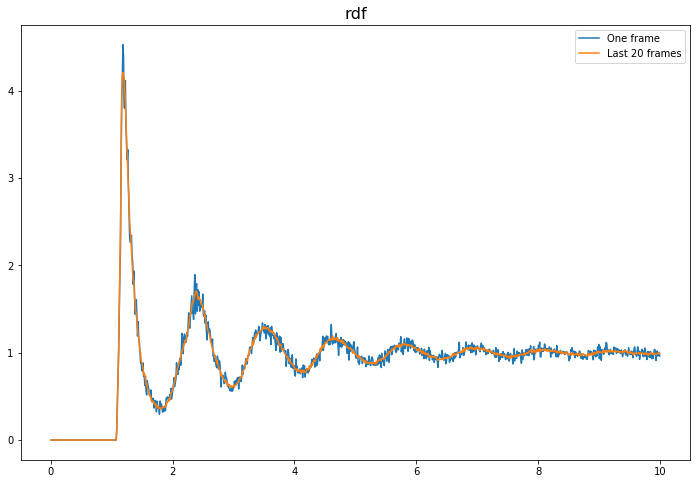# freud.density.RDF: Accumulating g(r) for a Fluid¶

The freud.density module is intended to compute a variety of quantities that relate spatial distributions of particles with other particles. This example demonstrates the calculation of the radial distribution function $$g(r)$$ for a fluid, averaged over multiple frames.

:

import numpy as np
import freud
import matplotlib.pyplot as plt

data_path = "data/phi065"

def plot_rdf(box_arr, points_arr, prop, r_max=10, bins=100, label=None, ax=None):
"""Helper function for plotting RDFs."""
if ax is None:
fig, ax = plt.subplots(1, 1, figsize=(12, 8))
ax.set_title(prop, fontsize=16)
rdf = freud.density.RDF(bins, r_max)
for box, points in zip(box_arr, points_arr):
rdf.compute(system=(box, points), reset=False)
if label is not None:
ax.plot(rdf.bin_centers, getattr(rdf, prop), label=label)
ax.legend()
else:
ax.plot(rdf.bin_centers, getattr(rdf, prop))
return ax


Here, we show the difference between the RDF of one frame and an accumulated (averaged) RDF from several frames. Including more frames makes the plot smoother.

:

# Compute the RDF for the last frame
box_arr = [box_data[-1].tolist()]
pos_arr = [pos_data[-1]]
ax = plot_rdf(box_arr, pos_arr, 'rdf', label='One frame')

# Compute the RDF for the last 20 frames
box_arr = [box.tolist() for box in box_data[-20:]]
pos_arr = pos_data[-20:]
ax = plot_rdf(box_arr, pos_arr, 'rdf', label='Last 20 frames', ax=ax)

plt.show()The difference between accumulate (which should be called on a series of frames) and compute (meant for a single frame) is more striking for smaller bin sizes, which are statistically noisier.

:

# Compute the RDF for the last frame
box_arr = [box_data[-1].tolist()]
pos_arr = [pos_data[-1]]
ax = plot_rdf(box_arr, pos_arr, 'rdf', bins=1000, label='One frame')

# Compute the RDF for the last 20 frames
box_arr = [box.tolist() for box in box_data[-20:]]
pos_arr = pos_data[-20:]
ax = plot_rdf(box_arr, pos_arr, 'rdf', bins=1000, label='Last 20 frames', ax=ax)

plt.show()